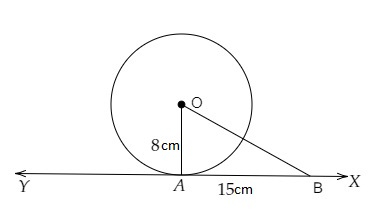# O is the centre of a circle of radius 8 cm. The tangent at a point A on the circle cuts a line through O at B such that $AB = 15\ cm$. Find OB.

Given:

O is the centre of a circle of radius 8 cm. The tangent at a point A on the circle cuts a line through O at B such that $AB = 15\ cm$.

To do:

We have to find the length of OB.

Solution:Radius $OA = 8\ cm$.

$AB = 15\ cm$

Let $XY$ be the tangent to the circle at $A$.

$OA\ perp\ XY$

In right angled triangle $OAB$,

By Pythagoras theorem,

$OB^2= OA^2+ AB^2$

$OB^2 = 8^2 + 15^2$

$= 64 + 225$

$= 289$

$= 17^2$

Therefore,

$OB = 17\ cm$

The length of OB is 17 cm.

Updated on: 10-Oct-2022

49 Views